# Introduction to Binary Tree: Properties, Types, Representation and Application

The tree is a non-linear data structure that stores data in the form of a hierarchical structure. It is made up of a finite set of elements called nodes. Good examples of a tree are data stored in a computer file system, hierarchical records of employees in a company, recursion tree structure, etc.

We can think tree as a recursive object, defined as a collection of smaller trees starting at a root node. That’s why we can solve many tree problems using recursion: Assuming we knew the solution to the smaller subtree, how could we combine it to get the final answer? Similarly, we can also think tree as a particular version of a directed acyclic graph (A graph with no cycle).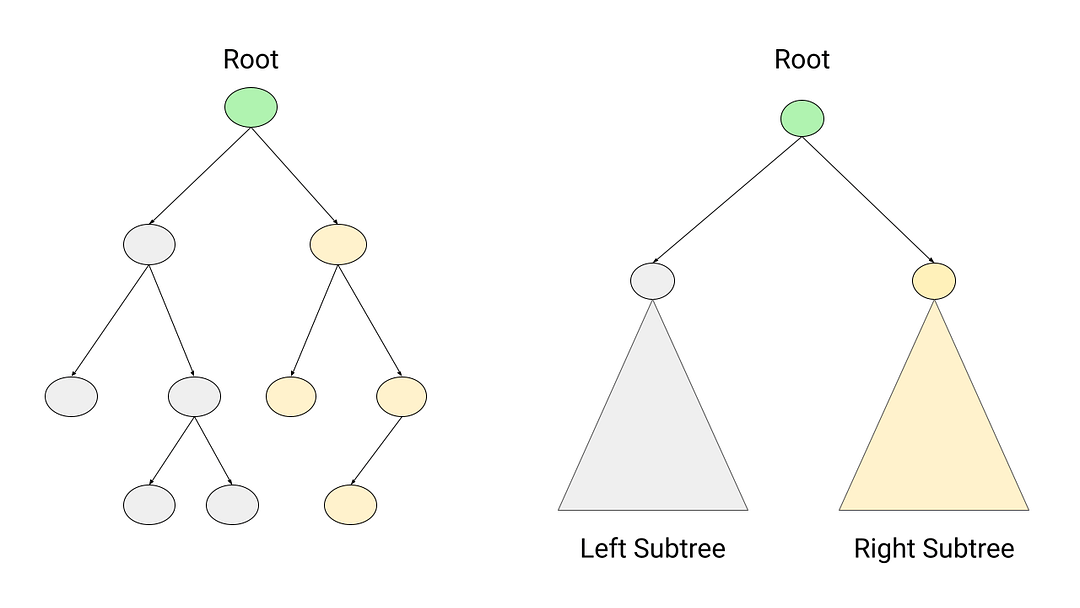### What is a Binary Tree?

Trees can have any number of children, but the simplest and most common type of tree is a binary tree, where each node has at most two child nodes (called left child and right child). In other words, a node in a binary tree can have 0 or 1 or 2 child nodes. So there are three fundamental components of a binary tree: root node, left sub-tree connected via a left pointer, and right sub-tree connected via right pointers. All three elements are disjoint, or they have no nodes in common.

• The node at the top of the tree is called the root node.
• A node having no child node is called a leaf node.
• A node with at least one child node is called an internal node.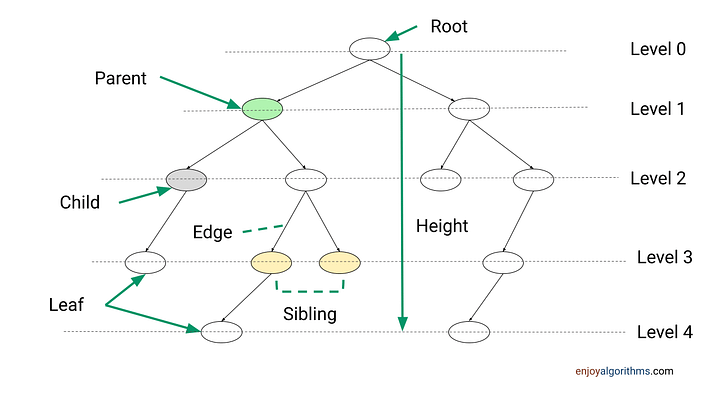### Terminologies in a Binary Tree

Node: An element in a tree that stores data and pointers to its children.

Size: Number of nodes in the tree.

Child and Parent: If node X is connected via the pointer of node Y, then X is a child of node Y, and Y is the parent of node X. In other words, a child node is an immediate descendant of a node, and a parent is an immediate predecessor of a node.

Siblings: If some pair of nodes X and Y have the same parent, then X and Y are called siblings.

Edge: The line that connects two nodes is known as an edge. If there are n nodes in a tree, there exist n - 1 edges.

Path: If n1,n2,…,nk is the hierarchy of nodes in the tree such that ni is the parent of ni + 1 for 1 ≤ i < k, then this sequence is called a path from n1 to nk, and the length of the path is k−1. There is exactly one path from the root node to any other node in the tree.

Height: The height of a node is the maximum number of edges between that node and a leaf node. The height of the tree is the height of the root node.

Level: The level of a node is defined as the height difference between that node and the root node (or the number of edges in the path from the root to that node). As a convention, we can consider the root node level to be 0.

Ancestor and Descendant: If there is a path from node X to node Y, then X is an ancestor of Y, and Y is a descendant of X. So all nodes in the tree are descendants of the root of the tree, while the root is the ancestor of all nodes.

### Types of Binary Trees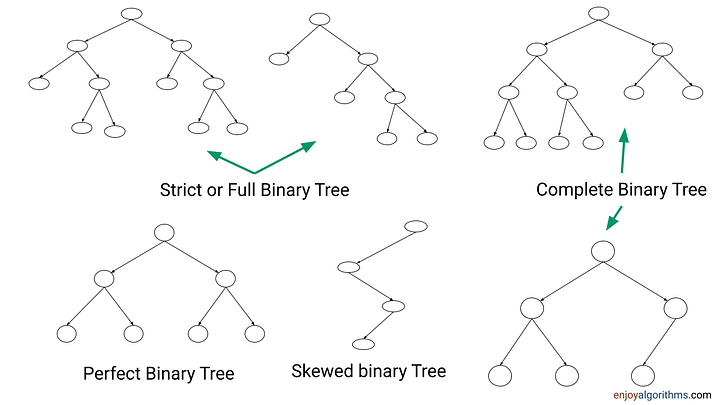#### Full Binary Tree or Strict Binary Tree

It is a tree in which each node has either zero or two child nodes. The Huffman coding and Segment tree are good examples of a full binary tree.

#### Complete Binary Tree

It is a tree in which all the levels are completely filled except the last level. And at the last level, all nodes are as much to the left as possible (from left to right). An excellent example of a complete binary tree is Heap.

#### Perfect Binary Tree

In a perfect binary tree, all internal nodes have 2 children, and all leaf nodes are on the same level. In other words, all levels in a complete tree are filled to their maximum capacity.

#### Height Balanced Binary Tree

A binary tree with average height O(log n) is called height-balanced, where n is the number of nodes. For example, the AVL tree and Red-black tree are height-balanced binary search trees, which ensure O(log n) time complexity for several operations like search, insert, delete, finding maximum, finding the minimum, etc.

#### Skewed Binary Tree

A skewed tree is one in which every internal node has one child. The height of such trees is O(n), which looks similar to the linked list in terms of performance.

#### Ordered Binary Tree

A tree in which every node follows some order property is called an ordered tree — for example, BST, Heap, Segment tree, etc.

### Some Important Binary Tree Properties

Binary Tree Property1: The total number of nodes in a perfect binary tree of height h = 2^(h+1) — 1. In other words, a perfect binary tree is a scenario of the maximum number of nodes.

Proof: At each level, the number of nodes is 2^l, where l represents a level. So the total number of nodes = 2^0 + 2^1 + 2^2 + … +2^h = 2^(h+1)-1 (Using the formula for the sum of geometric progression).

Binary Tree Property 2: The number of leaf nodes in a perfect binary tree of height h = 2^h. So total number of internal nodes = Total number of nodes — Total number of internal nodes = [2^(h+1) — 1] — 2^h = 2^h — 1. In other words, if the number of leaves in a full binary tree is n, then: The number of internal nodes = n — 1, The total number of nodes = n + n — 1 = 2n — 1

Binary Tree Property 3: A binary tree with n leaves has at least log n + 1 levels. Similarly, if a binary tree has n nodes, the minimum possible height or the minimum number of levels is log(n+1). Think!

Binary Tree Property 4: In a strict binary tree (every node has 0 or 2 children) with n leaf nodes, the number of internal nodes will be n — 1. In other words, the count of the leaf nodes (L) is always one more than the count of internal nodes (I), i.e., L = I + 1.

Proof using mathematical induction: Suppose the above property is true. Now we extend this tree and add 2 nodes to any of the leaf nodes because we cannot extend this tree in any other way without violating the conditions of a full binary tree. So, if we add 2 child nodes to any of the leaf nodes, that leaf node will become an internal node. So the number of leaf nodes increased by 1, and the number of internal nodes also increased by 1, maintaining the property L = I + 1 still holds true. Thus, this property will still hold true whenever we grow this tree to hold more nodes.

Binary Tree Property 5: In a Binary tree, the number of leaf nodes is always one more than nodes with two children i.e., L = I + 1, where L = Number of leaf nodes, and I = number of internal nodes with two children.

Proof: Suppose we define a degree of a node, which is equal to the total number of incoming or outgoing edges from a node. The sum of degrees in a binary tree = 2 * (The total number of edges). The idea is simple: every edge contributes degree 2 in a binary tree i.e. one for an outgoing edge from a node and one for an incoming edge to a node.

Equation 1: Suppose the total number of nodes with one child = K. So the total number of nodes in a binary tree = I + L + K.

Equation 2: As we know from the above discussion, The total number of edges =The total number of nodes — 1. So the sum of degrees in a binary tree = 2 * (The total number of nodes — 1) = 2 (I + L + K — 1)

Suppose the root has two children:

• The degree of the root = 2
• The degree of a leaf node = 1
• The degree of an internal node with one child = 2
• The degree of an internal node with two children = 3

Equation 3: The sum of degrees in a binary tree = The sum of degrees of nodes with two children except root + The sum of degrees of nodes with one child + The sum of degrees of leaves + Root’s degree = 3(I — 1) + 2* K + L + 2

Both equations 2 and 3 are the same.

=> 2 (I + L + K — 1) = 3(I — 1) + 2* K + L + 2

=> 2I + 2L + 2K — 2 = 3I — 3 + 2K + L + 2

=> L — 2 = I — 3 + 2

=> L = I + 1 (Proved)

Note: We can also follow a similar proof approach if the root has one child. In this case, the degree of the root will be 1.

### Representation of Binary Trees in Data Structure

There are two primary ways to represent binary trees in programming:

• Sequential Representation

#### Linked Representation of Binary Trees

In this representation, every node is an object of a class that stores three things: the value of the node, the pointer or reference to the left child, and the pointer or reference to the right child. In other words, every node store some data and contain the address of their children.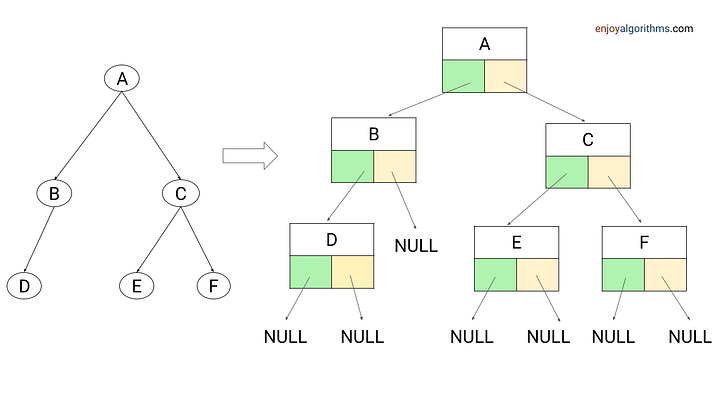``````class TreeNode
{
int val
TreeNode leftChild
TreeNode rightChild
}``````
• TreeNode is a class blueprint of the node object.
• val is the data stored inside the node. Based on requirements, we can also add some additional data fields inside the node (This is called augmentation which can be used to solve several tree problems).
• leftChild stores the pointer or reference to the left child of a node object. If there is no left child, then leftChild will be NULL.
• rightChild stores the pointer or reference to the right child of a node object. If there is no right child, then rightChild will be NULL.
• For the leaf node, the left and right child pointers will be NULL.

#### Sequential Representation of Binary Trees

The sequential representation of the binary tree is an array-based implementation where we store the value of the nodes at array indexes. The numbering or indexing of nodes can be done level-by-level from left to right, starting from 0 to n-1 (0-based indexing) or 1 to n (1-based indexing).

This indexing order is similar to the complete binary structure, where all levels except the bottom are filled completely, and the bottom level has all of its nodes filled in from left to right. So the complete binary tree of n nodes has only one possible shape.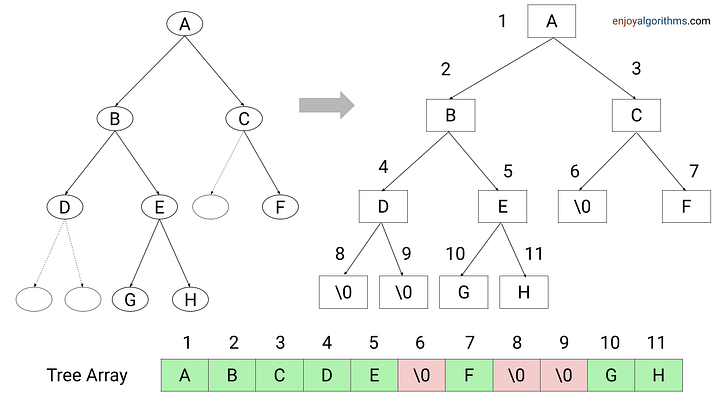But unlike linked representation, we need to define the size of the array in advance. To ensure the correct position and parent-child relationship, we need to allocate space for all possible node indexes. So the size of an array to represent a binary tree of height h is equal to the maximum number of nodes possible in a complete binary tree i.e. 2^(h+1) — 1. If a node is not present, it can be represented by some unique empty character.

As we can see, whether a node is present or not, memory is allocated for a complete binary tree of height h. So if there are a lot of holes present in the tree, the sequential representation might be a bad idea as it takes up a lot of space.

Based on the pattern of storing nodes within the array, we can derive a simple formula for calculating the parent, left, and right child of a node. No explicit pointers are required! For any node at index i:

1-based indexing: Parent(i) = ⌊i/2⌋, Left child(i) = 2i (if 2i < n), Right child(i) = 2i + 1 (if 2i + 2 < n)

0-based indexing: Parent(i) = ⌊(i-1)/2⌋, Left child(i) = 2i +1 (if 2i + 1 < n), Right child(i) = 2i + 2 (if 2i + 2 < n)

Note: In some situations, there is no or less memory overhead in the array implementation. The best example of this is the array implementation of the heap (complete binary tree structure) and segment trees (Strict binary tree structure).

### Applications of Binary Tree in Data Structures

• Binary tree: Storing hierarchical data like folder structure, organization structure, Document Object Model to represent documents, etc.
• Binary search tree: Used to perform efficient query and modification operations(Searching, insertion, deletion, etc.), To implement map and set objects in languages’ libraries.
• Trie data structure: Autocompletion feature, spell-checking, Contact search on a phone, swipe features in mobile keypad, Longest prefix matching, etc.
• Heap data structure: Used for efficient implementation of priority queues, process scheduling in operating systems, Dynamic memory allocation, Finding kth smallest or largest in an array, etc.
• Advanced data structures: Syntex parsing in the compiler(Syntax Tree), Wireless networking (Treap), Range query operations(Segment Tree, Fenwick tree), Finding the nearest neighbor to a particular point (K Dimensional Tree), To store data on the drive (B-tree), 3D computer graphics(BSP tree), Fast full-text search in word processors (Suffix tree)

### Blogs to explore further

Enjoy learning, Enjoy algorithms!

Share on social media:

© 2022 Code Algorithms Pvt. Ltd.